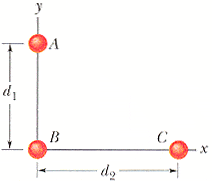# Superposition of 3 point masses

## Homework Statement

In the figure below, three 2.00 kg spheres are located at distances d1 = 0.300 m and d2 = 0.400 m. What are the magnitude and direction of the net gravitational force on sphere B due to spheres A and C?F = GMm/r^2

## The Attempt at a Solution

F=sqrt((4G/0.4^2)^2 + (4G/0.3^2)^2)
F = 3.0E-9

But that answer is wrong. Is there a mistake in my arithmetic? Of am i missing another term?

haruspex
Homework Helper
Gold Member
Is there a mistake in my arithmetic?

F (B->C) = G(2)(2)/(0.4^2) = 4G/0.16
F (B->A) = G(2)(2)/(0.3^2) = 4G/0.09

F (B->net) = F(B->C) + F(B->A)
Can't add these together since they aren't the same direction, so use Pythagoras' theorem:

F^2 (B->net) = F^2(B->C) + F^2(B->A) = sqrt((4G/0.16)^2 + (4G/0.09)^2)

haruspex
Homework Helper
Gold Member
F (B->C) = G(2)(2)/(0.4^2) = 4G/0.16
F (B->A) = G(2)(2)/(0.3^2) = 4G/0.09

F (B->net) = F(B->C) + F(B->A)
Can't add these together since they aren't the same direction, so use Pythagoras' theorem:

F^2 (B->net) = F^2(B->C) + F^2(B->A) = sqrt((4G/0.16)^2 + (4G/0.09)^2)
Yes, I understand you're doing that, but your result is inaccurate (not wildly wrong). I can't tell where the inaccuracy comes in unless you post all steps.

haruspex
Homework Helper
Gold Member
Just had a thought... is this what you meant to post, or have you left out a digit?
3.0E-9

Yes, I understand you're doing that, but your result is inaccurate (not wildly wrong). I can't tell where the inaccuracy comes in unless you post all steps.
Im sorry, I don;t understand what you mean by "Post all steps", these are all the steps I have done. What more is there?

Just had a thought... is this what you meant to post, or have you left out a digit?
3.0E-9 is the result my calculator gives me, although the numbers may be cut-off since the calculator display is limited to 10 digits. I'll use an online calculator and see if it makes a difference

3.0E-9 is the result my calculator gives me, although the numbers may be cut-off since the calculator display is limited to 10 digits. I'll use an online calculator and see if it makes a difference
wolfram alpha gives 3.40125... × 10^-9 , so that might be the problem. Can you verify that the way I did the question is indeed correct?

haruspex
Homework Helper
Gold Member
wolfram alpha gives 3.40125... × 10^-9 , so that might be the problem
d1= 0.3
d2= 0.4
1/d1^4= 123.46
1/d2^4= 39.06
sum= 162.52
sqrt(sum)= 12.75
G= 6.67E-011
m*m= 4
*G*m*m= 3.40E-009

d1= 0.3
d2= 0.4
1/d1^4= 123.46
1/d2^4= 39.06
sum= 162.52
sqrt(sum)= 12.75
G= 6.67E-011
m*m= 4
*G*m*m= 3.40E-009

3.40125 × 10^-9 is indeed the correct answer! It was my calculator that ran out of displaying digits to properly show the number. Thank you for your time.